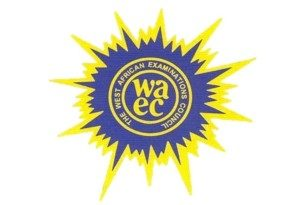## WAEC 2019 MATHEMATICS OBJ AND ESSAY VERIFIED ANSWER### (Mathematics obj and theory answer)

Use this very accurate.
Maths-Obj
11BCBCBAAACD
21DCCBCCABBA
31AABDDCDDCC
41BBCDCCBCCD

=======================

(4)
Since <PQR = <PRS = 90°
Using Pythagoras theorem
|PR|² = |PQ|² + |QR|²
|PR|² = 3² + 4²
|PR|² = 9 + 16
|PR|² = 25 PR = √25
|PR| = 5cm
Considering PRS
|PS|² = |PR|²+|SR|²
13² = 5² + |SR|²
169 = 25 + |SR|²
|SR|² = 169 – 25
|SR|² = 144
|SR| = √144 = 12cm

Hence the area of the quadrilateral = Area of triangle PQR + area of PRS
= 1/2bh + 1/2bh
= 1/2×4×3 + 1/2×12×5
= 6+30 = 36cm

(4)
======================================

(3a)
Total ratio =3+5+8=16
Ali’s share =3/16 * 420,000/1
=210,000
Son’s of Ali and Yusuf’s share = N78,750+ N210,000
= N288,750

(3b)
2(1/8)^x=32^x-1
2^1(2^-3)^x=2^5(x-1)
2^1 * 2^-3x=2^5(x-1)
2^-3x+1=2^5(x-1)

-3x+1=5(x-1)
-3x+1=5x-5
-5x-3x= -5-1
-8x/-8= -6/-8
x=3/4

(3)
======================================

(1a)
110ₓ = 40₅
change all to base ten.
110ₓ = 40₅

1x²+1x ¹+0x⁰ = 45¹+0*5⁰
x² + x + 0 = 20
x² + x – 20 = 0

x² + 5x – 4x – 20 = 0
x(x+5) – 4(x+5) = 0
(x-4)(x+5) = 0
Either x-4 = 0 or x + 5 = 0
x = -5 or 4
therefore, x= 4

(1b)
15/√75 + √108 + √432
= 15/√253 + √363 + √144*3
= 15/5√3 + 6√3 + 12√3
= 3/√3 + 18√3
= 3√3/3 = 18√3
= √3 + 18√3
=19√3

(1)
======================================

(5a)
No of red balls = 3
No of green balls = 5
No of blue balls = x
Prob.(red ball) = no of total outcome/no of possible outcome
Pr(red) = 3/3+5+x = 1/6
3/8+x = 1/6
6(3) = 1(8+x)
18 = 8 + x
X = 18 – 8 = 10
Therefore the no of blue ball = 10

(5b)
Probability of picking a green ball
P(g) = no of green balls/no of possible outcome
P(g) = 5/3+5+10 = 5/18
=5/18

(5)
======================================

(2a) y2-y1/x2-x1 = y-y1/x-x1

Given : A(-2,7) and B(2,-3)
-3-7/2+2 = y-7/x+2
-10/4=y-7/x+2
4(y-7)= -10(x+2)
4y-28= -10x-20
4y= -10x+28-20
4y= -10x+8
2y= -5x+4
OR
5x+2y=4

(2b)
5b-a/8b+3a=1/5
(5b-a)/b/(8b+3a)/b =1/5
5-(a/b)/8+3(a/b) =1/5
8+3(a/b)= 5 [ 5-(a/b)]
8+3(a/b)=25-5(a/b)
3(a/b) + 5(a/b)=25-8
8(a/b)=17
a/b=17/8

(2)
======================================

(6ai)
F α M1M2/d²
F = KM1M2/d²
Given F = 20N, M1= 25kg, M2 = 10kg and d = 5m
20 = k(25)(10)/5²
250k = 500
k = 500/250 = 2
Expression is
F = 2M1M2/d²

(6aii)
Making d subject
d = √2M1M2/F
d = √2 ×7.5×4/30
d = √60/30 = √2
d = √2m or 1.41m

(6b)
Draw the diagram
X+X+60+X+80+X+40+X+20 = 540(sum of angles in a Pentagon)
5x + 200 = 540
5x = 540 – 200
5x = 340
X = 340/5
X = 68

(6)
======================================

(8a)
1/3x – 1/4(x+2)>_ 3x -1⅓
1/3x – 1/4(x+2)>_3x – 4/3
Multiply through by the L. C. M(12), we have
4x – 3(x + 2)>_36x – 16
4x – 3x – 6 >_ 36x – 16
-6+16 >_36x + 3x – 4x
10 >_ 35x
35x _< 10
X = 10/35
X = 2/7

(8bi)
Draw the triangle
|AB|/66 = sin35
|AB| = 66sin35 = 66×0.5736 = 37.8576

Draw the right angled triangle
|AD| = 37.8576 × Tan52° = 37.8576 × 1.2799 = 48.45m
Height of tower = 48.45m

(8bii)
|AC|/66 = Cos35°
|AC| = 66 x cos35°
= 66 x 0.8192
= 54.0672

Tan = 41.86°
Angle of elevation of top of tower from c = 41.85°

(8)
======================================

(12a)
Given : siny = 8/17
Draw the right angle
From Pythagorean triple, third side is 15
Draw the right angle triangle
tan y = 8/15

tan y/1+2tany = 8/15/1+2(8/15) = 8/15/1+16/15

tany /1+2tan y = 8/31

(12b)
Amount shared = #300,000
Otobo’s share = #60,000
Ade’s share = 5/12 × #(300,000-60,000)
= 5/12 × #240,000
=#100,000

Adeobi’s share = #300,000 – (#60,000 + #100,000)
= 300,000 – 160,000
=#140,000

CHECK OUT:  WAEC 2019 FINANCIAL ACCOUNTING OBJ AND ESSAY VERIFIED ANSWER

60,000 : 100,000 : 140,000
60 : 100 : 140
6 : 10 : 14
3 : 5 : 7

(12)
======================================

(11ai)
ar² = 1/4 ……(1)
ar^5= 1/32 …..(2)
Divide eqn (2) by eqn(1)
ar^5/ar² = 1/32÷1/4
r³ = 1/32 × 4/1
r³= 1/8
r³ = 2-³
r = 2-¹
r = 1/2
Common ratio = 1/2
Put this into eqn (1)
a(1/2)² = 1/4
a(1/4) = 1/4
a = (1/4)/(1/4) = 1
First term, a = 1

(11aii)
Seventh term, T7 = ar^6
=(1)(1/2)^6
=1/64

(11b)
Given : X = 2 and X = -3
(X – 2)(X + 3) = 0
X² + 3x – 2x – 6 , 0
X² + x – 6 = 0
Comparing with ax²+bx+c = 0
a = 1
b = 1
C = -6

(11)
======================================

(10)
130kg of tomatoes for #52,000
Half of the tomatoes
130/2 = 65kg sold at 30%
Profit = #52,000/2 = 26,000
#26,000 = 100%
X = 130%
X = 26000 × 130/100
= #33,800

Then 65kg was then sold at reduction of 12% per kg
Recall that the initial cost price = 52000/130
=400kg
65kg sold at = 33,000/65
=#520/kg
Then for 12% reduction
520 × 88/100 = 457.6/kg
(a)
The new selling price per kg = #457.6/kg

(b) 65kg – 5kg = 60kg
(60kg×457.6kg)+33,800
= #61,256.00

#profit = selling price /cost price × 1000/1
=61256/52000×100/1= 117.8
= 17.8%

(10)
======================================

(13a)
T^0S=2TU^0 (angle at centre = 2 time angle at circumstance)
TOs=2*68°=136°

Now RT^0+RS^0+T^0S+SRT^0=360°(sum of angle in a quadrilateral)
90° + 90° + 136° + x = 360°
X+316°=360°
X=360°-316
X=44°

(13b)
Let tank B hold x litres
; Tank A hold (x+600)literally
3(x-100)=(x+600-100)
3x-300=x+500
3x-x=500+300
2x=800
X=800/2=400
Tank B holds 400 litres
Tank A holds (400+600)=1000litres

(13)
==============================

Gce whatsapp exam runs,
Neco whatsapp free exam runs,Join Our Telegram Channel |Whatsapp Our Admins: Click Any Name Mr.Paul or Mr.Henry